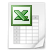### Symmetric transformation (Liki)

Paulus Gerdes introduced the Liki magic square (see http://plus.maths.org/content/new-designs-africa). He showed that it is possible to transform a square with consecutive numbers into a magic square by swapping half of the numbers symmetrically. You can use this method to construct magic squares which are a multiple of 4 (= 4x4, 8x8, 12x12, 16x16, ... magic square).

The 4x4 magic square contains the numbers 1 up to 16. Each number has an inverse number. The inverse number is the largest number of the square plus 1 minus the original number. See below the results:

1   --> Inverse number is 16 + 1 -/- 1 =
16
2   --> Inverse number is 16 + 1 -/- 2 =
15
3   --> Inverse number is 16 + 1 -/- 3 =
14
4   --> Inverse number is 16 + 1 -/- 4 =
13
5   --> Inverse number is 16 + 1 -/- 5 =
12
6   --> Inverse number is 16 + 1 -/- 6 =
11
7   --> Inverse number is 16 + 1 -/- 7 =
10
8   --> Inverse number is 16 + 1 -/-   8 =
9
9   --> Inverse number is 16 + 1 -/-   9 =
8
10 --> Inverse number is 16 + 1 -/- 10 =
7
11 --> Inverse number is 16 + 1 -/- 11 =
6
12 --> Inverse number is 16 + 1 -/- 12 =
5
13 --> Inverse number is 16 + 1 -/- 13 =
4
14 --> Inverse number is 16 + 1 -/- 14 =
3
15 --> Inverse number is 16 + 1 -/- 15 =
2
16 --> Inverse number is 16 + 1 -/- 16 =
1

Transform the 4x4 square with consecutive numbers in a symmetric 4x4 magic square:

4x4 square with consecutive numbers

 28 32 36 40 34 34 10 1 2 3 4 26 5 6 7 8 42 9 10 11 12 58 13 14 15 16

Note that the square with consecutive numbers is already symmetric and the (main) diagonals give already the magic sum of 34.

Symmetric 4x4 magic square (1)

 34 34 34 34 34 34 34 1 15 14 4 34 12 6 7 9 34 8 10 11 5 34 13 3 2 16

ór

Symmetric 4x4 magic square (2)

 34 34 34 34 34 34 34 16 2 3 13 34 5 11 10 8 34 9 7 6 12 34 4 14 15 1

Use this method to construct magic squares of order is multiple of 4 from 4x4 to infinity. See 4x48x812x1216x1620x2024x2428x2832x324x4, symmetric transformation (Liki).xls

Take one step extra and you can transform a 4x4 square with consecutive numbers into the famous square of Albrecht Dürer:

Dürer’s magic square:

 28 32 36 40 28 36 32 40 34 34 34 34 34 34 34 34 34 34 10 1 2 3 4 10 1 3 2 4 34 16 3 2 13 26 5 6 7 8 26 5 7 6 8 34 5 10 11 8 42 9 10 11 12 42 9 11 10 12 34 9 6 7 12 58 13 14 15 16 58 13 15 14 16 34 4 15 14 14x4, Dürer transformation.xls